# Numerical Differentiation

Problem

You have a table of experimentally obtained data representing some function and you'd like to approximate the derivatives of that function.

Solution

Discussion

A well-respected professor once told me that numerical differentiation is death. That's a pretty strong statement, and what he meant was that once you start taking finite differences (a way to approximate derivatives numerically), accuracy goes downhill fast, ruining your results. This is because numerical differentiation can be very inaccurate due to its high sensitivity to inaccuracies in the values of the function being differentiated. This is in contrast to numerical integration, which is far more insensitive to functional inaccuracies because it has a smoothing effect that diminishes the effect of inaccuracies in the values of the function being integrated.

If you have an analytic form of the function under consideration, then you're far better off deriving an analytic expression for the derivative, as opposed to resorting to numerical differentiation. On the other hand, if you have experimentally obtained data with no analytic expression for the function, then you may have to resort to numerical differentiation. This latter case is the one we'll consider here.

By definition, the derivative of a function is: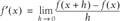When given tabulated data representing a function, we don't have the luxury of allowing h to go to zero. In this case, we have the function evaluated at some point x and some other point x + dx that's a finite distance, dx, away from x. We can approximate the derivative of a function using so-called finite differences, where we have: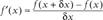It then follows that as dx gets smaller, this approximation should become more accurate. The trouble with tabulated data is that often dx is not as small as we'd like it to be for purposes of approximating derivatives. That said, we can use this approach to at least approximate the derivative of a function. The question then arises: when dealing with a tabulated set of data of the form (xi, yi), what set of values do you use to approximate the derivative at point i? Specifically, do you use a forward difference, (f(xi +1) - f(xi))/dx, or a backward difference, (f(xi) - f(xi -1))/dx? The answer is that you could use either approach or better yet, neither. An even better approach uses a so-called central difference, (f(xi +1) - f(xi -1))/(2dx).

Let's consider each of these in the context of an example using a known analytic function so we can compare the approximate results to known theoretical results. Consider the function f(x) = x3. The derivative of this function is easily derived and is f'(x) = 3x2. Figure 10-6 shows tabulated values for this function and its theoretical derivative over the range x = [0,1].

I've also included several columns corresponding to approximations of the function's derivative using several different approaches, namely, forward differences, backward differences, central differences, and a so-called 5-point formula, which I'll come back to shortly.

Figure 10-6. Comparison of finite differencing schemesThe formulas in column F for the forward difference approximation are of the form =(D5-D4)/\$G\$1, where the difference D5-D4 is the forward difference in y-values and G1 contains the value for dx (labeled h in the spreadsheet). Similarly, the formulas in column G for the backward difference approximation are of the form =(D5-D4)/\$G\$1. The central difference formulas in column H are of the form =(D6-D4)/(2*\$G\$1).

Plotting these results along with the theoretical derivatives yields the chart shown in Figure 10-7.

The dy/dx curve is the theoretical derivative. The others are the various approximations. Clearly, the central difference approach yields far better results than either forward differencing or backward differencing. This is true in general, and central differences are usually preferred over the other two approaches. For this particular function, the central difference approximation curve lies directly on top of the theoretical curve, implying very close agreement. You can also see this from the tabulated results shown in Figure 10-6.

The central difference approach has the effect of smoothing the derivative at a point as it considers the values of the function both ahead of and behind the point under consideration. The 5-point formula mentioned earlier extends this idea, taking into account even

Figure 10-7. Chart comparing approximate to theoretical results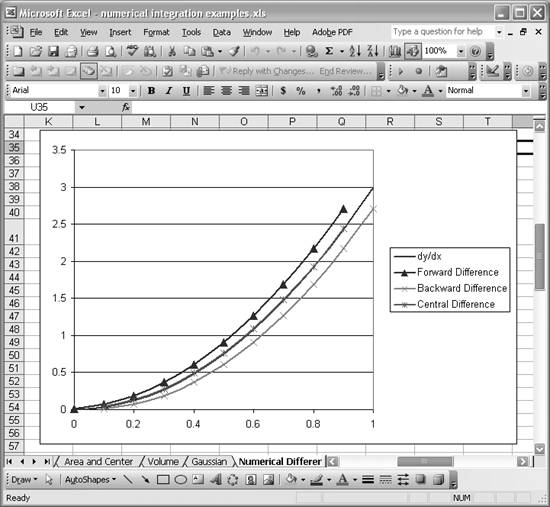more points around the specific point under consideration. The 5-point formula is commonly presented in texts on numerical methods and the formula is as follows:In this example, the 5-point formula is of the form =(1/(12*\$G\$1))*(D4-8*D5+8*D7-D8). This formula corresponds to the approximation in cell I6.

For this particular example, the 5-point formula yields results exactly matching the theoretical derivatives. In practice, this isn't always the case, but it does show how much better the 5-point formula is than forward or backward differencing for this example.

As mentioned earlier, numerical differentiation is very sensitive to errors in the values of the function being considered, and this is one of the main sources of error in numerical derivatives. The example so far has included exact values for the function under consideration.

In practice, if this data was measured experimentally, you'd expect some error in values.To simulate such error, I computed some random deviations for the example function. Figure 10-8 shows the simulated experimental data plotted against the theoretical values from the function under consideration.

Figure 10-8. Simulated experimental data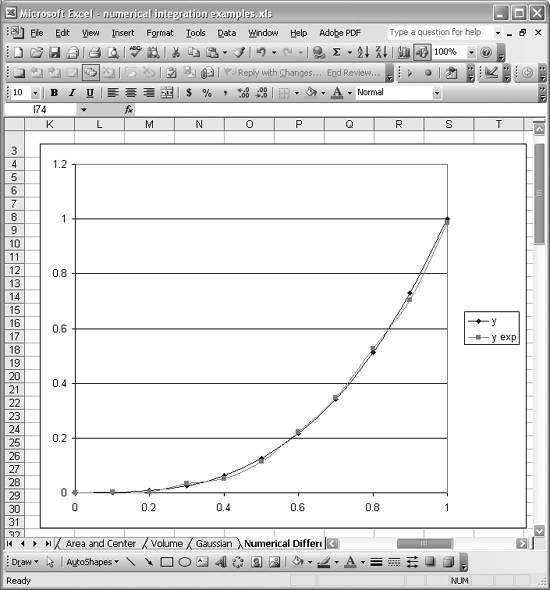As you can see, the curve labeled y exp fairly closely approximates the theoretical curve of y = x3, but with some small errors. Now, let's look at the effect of these small errors on the derivatives as approximated using the numerical differentiation formulas shown earlier. Figure 10-9 shows the results.

This chart is dramatically different from that shown in Figure 10-7. Look at how wildly the forward and backward difference curves deviate from the curves shown in Figure 10-7. This is characteristic of numerical derivatives in light of small errors in the values of the function under consideration. Any small errors in the values of the function from point to point are amplified in the numerically obtained derivative.

These results are motivation for using the central difference or 5-point formulas, as they tend to smooth out these oscillations by considering more neighboring values of

Figure 10-9. Approximate derivatives for experimental data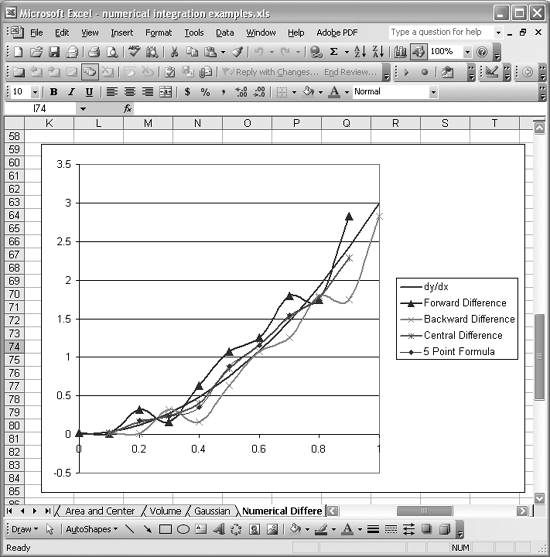the function. Figure 10-9 illustrates that these latter methods do indeed yield far better approximations. The curves of the derivative using these two approaches still aren't as smooth as the theoretical curve, but they are far better than either the forward or backward difference curves.

The smoothing effect offered by formulas like the central difference and 5-point formulas has inspired other techniques for approximating derivatives. Indeed, it would seem plausible to smooth the tabulated functional values before computing numerical derivatives in an effort to increase accuracy. Moreover, you can fit an interpolation function to a given set of data and then use the derived analytic expression for the fit curve to derive an expression for the derivative, thus giving much smoother results for the derivative. (See Chapter 8 to learn how to fit equations in Excel.) In fact, this is the basis for many numerical methods that require derivative computations.

Another approach is to compute the numerical derivatives as discussed here and then fit a curve through those results to yield a smooth derivative curve. Figure 10-10 shows the central difference results from Figure 10-9 plotted along with a second-order least-squares fit polynomial. This polynomial was fit using Excel's trendline feature (see Chapter 8) on the central difference curve.

Figure 10-10. Smoothed derivative curve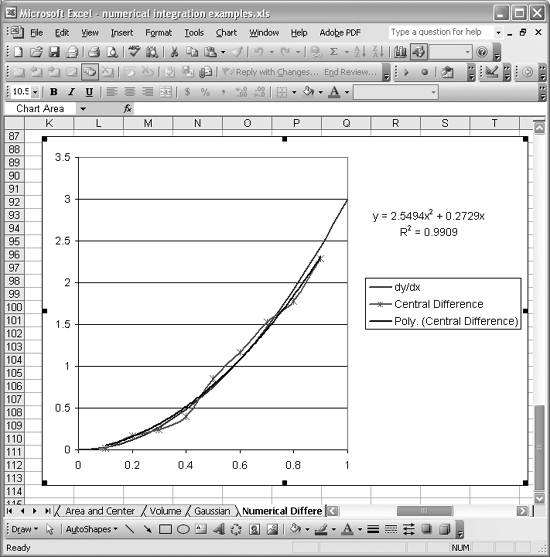The resulting trendline is smooth and approximates the theoretical derivative fairly well, giving a much better picture of the derivative than the raw derivative curve computed using central differences.

There are many schemes and formulas for computing numerical derivatives besides the ones discussed here. Further, you can apply these techniques to compute higher derivatives such as the second and third derivatives of a function. Such formulas are widely published in books on numerical methods, which include numerical differentiation.

Take a look at Chapter 8 to learn how to fit curves to data using Excel. You can use those fit curves to approximate derivatives as discussed here.Excel Scientific and Engineering Cookbook (Cookbooks (OReilly))
ISBN: 0596008791
EAN: 2147483647
Year: N/A
Pages: 206
Authors: David M Bourg